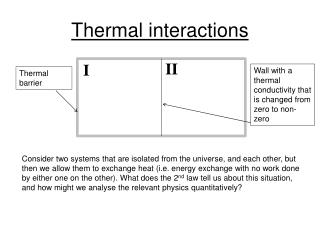Download PresentationThermal interactions

Thermal interactions - PowerPoint PPT Presentation

Thermal interactions. II. I. Wall with a thermal conductivity that is changed from zero to non-zero. Thermal barrier.I am the owner, or an agent authorized to act on behalf of the owner, of the copyrighted work described.
Download PresentationThermal interactions

Download Policy: Content on the Website is provided to you AS IS for your information and personal use and may not be sold / licensed / shared on other websites without getting consent from its author.While downloading, if for some reason you are not able to download a presentation, the publisher may have deleted the file from their server.

- - - - - - - - - - - - - - - - - - - - - - - - - - E N D - - - - - - - - - - - - - - - - - - - - - - - - - -
Presentation Transcript
1. Thermal interactions II I Wall with a thermal conductivity that is changed from zero to non-zero Thermal barrier Consider two systems that are isolated from the universe, and each other, but then we allow them to exchange heat (i.e. energy exchange with no work done by either one on the other). What does the 2nd law tell us about this situation, and how might we analyse the relevant physics quantitatively?

2. Thermal interactions II I Wall with a thermal conductivity that is changed from zero to non-zero Thermal barrier Consider two systems that are isolated from the universe, and each other, but then we allow them to exchange heat (i.e. energy exchange with no work done by either one on the other). What does the 2nd law tell us about this situation, and how might we analyse the relevant physics quantitatively? The system evolves to the macrostate (i.e. division of energy between the two systems, since that is all we are allowing to change) of maximum multiplicity and stays there. (dW/dEI)V,E,VI= 0; what is the condition on the invidual subsystems that assures this mathematical result?

3. Lecture 6-- CALM • What is the microscopic significance of an object’s heat capacity? • Something to do with time scales (3 answers) • Something to do with Heat Exchange (4 answers) • Something to do with multiplicity (8 answers) • This is not an easy question, but there are two key points to remember: • Knowing Cv(T) allows one to compute the change in entropy (i.e. the multiplicative change in number of microstates)! • It gives us some knowledge of the second derivative of the energy with respect to entropy (while holding external parameters fixed, e.g. V)

4. Chapter 3 problems (examples) Suppose we use it as an air conditioner for a room in your house:

5. Lecture 8– The importance of being slow Po P1 Fo F1 Let the gas push the piston to make the large volume, larger V is compensated by a decrease in E to keep the entropy constant! S1=So

6. Lecture 8– The importance of being slow Po P1 Fo F1 V1 Let the gas push the piston to make the large volume, larger V is compensated by a decrease in E to keep the entropy constant! S1=So Po P2 Vo V2 Alternatively, just let “Scotty” beam the barrier up to the enterprise and have the gas double its volume without doing work. S goes up by N ln(2)

7. Lecture 8– Typical Heat Engine

8. Lecture 8– Otto Cycle (simplified) Isochoric (constant volume) heating and cooling, connected by adiabatic expansions and compressions. The Internal combustion engine (ICE) is approximated by the Otto Cycle (not really closely, since in the ICE, the working fluid is not contained)